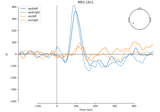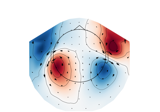# mne.viz.plot_arrowmap#

mne.viz.plot_arrowmap(data, info_from, info_to=None, scale=3e-10, vlim=(None, None), cnorm=None, cmap=None, sensors=True, res=64, axes=None, show_names=False, mask=None, mask_params=None, outlines='head', contours=6, image_interp='cubic', show=True, onselect=None, extrapolate='auto', sphere=None)[source]#

Plot arrow map.

Compute arrowmaps, based upon the Hosaka-Cohen transformation 1, these arrows represents an estimation of the current flow underneath the MEG sensors. They are a poor man’s MNE.

Since planar gradiometers takes gradients along latitude and longitude, they need to be projected to the flattened manifold span by magnetometer or radial gradiometers before taking the gradients in the 2D Cartesian coordinate system for visualization on the 2D topoplot. You can use the `info_from` and `info_to` parameters to interpolate from gradiometer data to magnetometer data.

Parameters
data`array`, shape (n_channels,)

The data values to plot.

info_frominstance of `Info`

The measurement info from data to interpolate from.

info_toinstance of `Info` | `None`

The measurement info to interpolate to. If None, it is assumed to be the same as info_from.

scale`float`, default 3e-10

To scale the arrows.

vlim`tuple` of length 2

Colormap limits to use. If a `tuple` of floats, specifies the lower and upper bounds of the colormap (in that order); providing `None` for either entry will set the corresponding boundary at the min/max of the data. Defaults to `(None, None)`.

New in v1.2.

cnorm

How to normalize the colormap. If `None`, standard linear normalization is performed. If not `None`, `vmin` and `vmax` will be ignored. See Matplotlib docs for more details on colormap normalization, and the ERDs example for an example of its use.

New in v1.2.

cmapmatplotlib colormap | `None`

Colormap to use. If None, ‘Reds’ is used for all positive data, otherwise defaults to ‘RdBu_r’.

sensors

Whether to add markers for sensor locations. If `str`, should be a valid matplotlib format string (e.g., `'r+'` for red plusses, see the Notes section of `plot()`). If `True` (the default), black circles will be used.

res`int`

The resolution of the topomap image (number of pixels along each side).

axesinstance of `Axes` | `None`

The axes to plot to. If `None`, a new `Figure` will be created. Default is `None`.

show_names

If `True`, show channel names next to each sensor marker. If callable, channel names will be formatted using the callable; e.g., to delete the prefix ‘MEG ‘ from all channel names, pass the function `lambda x: x.replace('MEG ', '')`. If `mask` is not `None`, only non-masked sensor names will be shown. If `True`, a list of names must be provided (see `names` keyword).

mask`ndarray` of `bool`, shape (n_channels,) | `None`

Array indicating channel(s) to highlight with a distinct plotting style. Array elements set to `True` will be plotted with the parameters given in `mask_params`. Defaults to `None`, equivalent to an array of all `False` elements.

Additional plotting parameters for plotting significant sensors. Default (None) equals:

```dict(marker='o', markerfacecolor='w', markeredgecolor='k',
linewidth=0, markersize=4)
```
outlines‘head’ | `dict` | `None`

The outlines to be drawn. If ‘head’, the default head scheme will be drawn. If dict, each key refers to a tuple of x and y positions, the values in ‘mask_pos’ will serve as image mask. Alternatively, a matplotlib patch object can be passed for advanced masking options, either directly or as a function that returns patches (required for multi-axis plots). If None, nothing will be drawn. Defaults to ‘head’.

contours

The number of contour lines to draw. If `0`, no contours will be drawn. If a positive integer, that number of contour levels are chosen using the matplotlib tick locator (may sometimes be inaccurate, use array for accuracy). If array-like, the array values are used as the contour levels. The values should be in µV for EEG, fT for magnetometers and fT/m for gradiometers. If `colorbar=True`, the colorbar will have ticks corresponding to the contour levels. Default is `6`.

image_interp`str`

The image interpolation to be used. Options are `'cubic'` (default) to use `scipy.interpolate.CloughTocher2DInterpolator`, `'nearest'` to use `scipy.spatial.Voronoi` or `'linear'` to use `scipy.interpolate.LinearNDInterpolator`.

show`bool`

Show the figure if `True`.

onselect

Handle for a function that is called when the user selects a set of channels by rectangle selection (matplotlib `RectangleSelector`). If None interactive selection is disabled. Defaults to None.

extrapolate`str`

Options:

• `'box'`

Extrapolate to four points placed to form a square encompassing all data points, where each side of the square is three times the range of the data in the respective dimension.

• `'local'` (default for MEG sensors)

Extrapolate only to nearby points (approximately to points closer than median inter-electrode distance). This will also set the mask to be polygonal based on the convex hull of the sensors.

• `'head'` (default for non-MEG sensors)

Extrapolate out to the edges of the clipping circle. This will be on the head circle when the sensors are contained within the head circle, but it can extend beyond the head when sensors are plotted outside the head circle.

New in v0.18.

Changed in version 0.21:

• The default was changed to `'local'` for MEG sensors.

• `'local'` was changed to use a convex hull mask

• `'head'` was changed to extrapolate out to the clipping circle.

sphere`float` | array_like | instance of `ConductorModel` | `None` | ‘auto’ | ‘eeglab’

The sphere parameters to use for the head outline. Can be array-like of shape (4,) to give the X/Y/Z origin and radius in meters, or a single float to give just the radius (origin assumed 0, 0, 0). Can also be an instance of a spherical `ConductorModel` to use the origin and radius from that object. If `'auto'` the sphere is fit to digitization points. If `'eeglab'` the head circle is defined by EEG electrodes `'Fpz'`, `'Oz'`, `'T7'`, and `'T8'` (if `'Fpz'` is not present, it will be approximated from the coordinates of `'Oz'`). `None` (the default) is equivalent to `'auto'` when enough extra digitization points are available, and (0, 0, 0, 0.095) otherwise.

New in v0.20.

Changed in version 1.1: Added `'eeglab'` option.

Returns
fig`matplotlib.figure.Figure`

The Figure of the plot.

Notes

New in v0.17.

References

1

David Cohen and Hidehiro Hosaka. Part II magnetic field produced by a current dipole. Journal of Electrocardiology, 9(4):409–417, 1976. doi:10.1016/S0022-0736(76)80041-6.

## Examples using `mne.viz.plot_arrowmap`#Visualizing Evoked data

Visualizing Evoked dataPlotting topographic arrowmaps of evoked data

Plotting topographic arrowmaps of evoked data